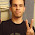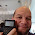## Thursday, May 10, 2012

### Calculus Revisited #19: Tests of Convergence

Welcome to Part 19 of our 21 Part series: Calculus Revisited. We are in the middle of working with series. Today's session: Tests of Convergence.

In a calculus course, you are often asked if certain series converge - that is, does the series have a sum. You are not necessarily asked to find the sum itself, just to say if the series has one.

Hint: This is good to know, the p-series.

For a series

∑ 1/n^p
n = 1

this series converges when p > 1 and diverges when p ≤ 1.

Now for the tests:

Comparison Test:

Give the infinite series ∑ a(n), find another comparable infinite series ∑ b(n). The series used for comparison dominates the series in question. Or:

b_n ≥ a_n for each n an integer

In addition, all terms both series, ∑ a(n) and ∑ b(n), are positive.

If ∑ b(n) converges, so does ∑ a(n).

However, if ∑ a(n) diverges, so does ∑ b(n).

There is a variant of the comparison test call the limit test. That is if

lim ( a_n / b_n) = L as n → ∞,

If ∑ b(n) converges and L < ∞, ∑ a(n) converges.
If ∑ b(n) diverges and L > 0, ∑ a(n) diverges.

Ratio Test:

For a given infinite series ∑ a(n), if

lim | a_n+1 / a_n | = L as n → ∞, and

L < 1,

then ∑ a(n) is absolutely convergent. If L = 1, the test is inconclusive. If L > 1, the series diverges.

Note: A series is absolutely convergent if

∑ |a(n)| = | a(1) | + | a(2) | + | a(3) | + ....

is convergent.

If ∑ a(n) converges but ∑ | a(n) | does not, then the series is said to be conditionally convergent.

Root Test:

For a given infinite series ∑ a(n), if

lim | a_n |^(1/n) = L as n → ∞, and

L < 1,

then ∑ a(n) is absolutely convergent. If L = 1, the test is inconclusive. If L > 1, the series diverges.

Alternating Series Test:

For the existing series:

∑ (-1)^n * a(n),

if the sequence {a_n} (ignoring the (-1)^n) is a strictly decreasing sequence of positive numbers (that is a_n+1 < a_n for all n), and

lim a_n = 0 as n → ∞,

the the alternating series is (at least) conditionally convergent.

Problems

1. Does this series converge?

∑ n^2 / (2n - 1)!
n = 1

Use the ratio test. Then:

a_n = n^2 / (2n - 1)!

a_n+1 = (n + 1)^2 / (2(n + 1) - 1)! = (n + 1)^2 / (2n + 1)!

a_n+1 / a_n
= (n + 1)^2 / (2n + 1)! * (2n - 1)! / n^2
= (n + 1)^2 / (n^2 * (2n + 1)(2n))
= (n^2 + 2n + 1) / (4n^4 + 2n^3)

As n → ∞, a_n+1 / a_n → 0 < 1.

By the ratio test, this series converges.

Sometimes reasoning will be necessary.

2. Does this series converge?

∑ 1 / (n * n^(1/n))
n = 1

Note that:
1 / (n * n^(1/n))
= 1 / (n^1 * n^(1/n))
= 1 / (n^(1 + 1/n))

Compare the series to the harmonic series:

∑ 1/n
n = 1

where 1/n ≥ 1/(n^(1 + 1/n))

By Limit test:

lim ( 1/(n^(1 + 1/n)) / (1/n) ) as n → ∞
= lim ( n / n^(1+ 1/n) ) as n → ∞

As n grows large, 1 + 1/n → 1
Hence, n / n^(1 + 1/n) → n / n → 1

Since ∑ (1/n) diverges, so does ∑ 1/ (n * n^(1/n))

3. Does this series converge?

∑ 1/(4n - 3)
n = 1

Use the limit test. Compare it to the harmonic series, which is known to diverge, and:

1/n ≥ 1/(4n - 3)

lim ( (1/(4n - 3)) / (1/n) ) as → ∞
= lim ( n / (4n - 3) ) as → ∞
= 1/4 > 0

Hence, the series in question diverges.

4. Does this series converge?

1 - 1/√3 + 1/√5 - 1/√7 + .... + (-1)^n/√(2n+1) + ....

Use the Alternating Series test.

Let a_n = 1/√(2n+1)

First a_n strictly decreases. Second, a_n → 0 as n → ∞.

By alternating series, the series conditionally convergent.

5. Does this series converge?

1/(2 ln 2) - 1/(3 ln 3) + 1/(4 ln 4) - 1/(5 ln 5) + ... + (-1)^n/(n ln n) + ....

Use the Alternating Series Test

Let a_n = 1/(n ln n),

a_n strictly decreases and a_n → 0 as n → ∞.

By alternating series, the series is conditionally convergent.

Next time we work with Taylor Series.

See you soon!

Eddie

This blog is property of Edward Shore. © 2012

1.You forgot about the integral test for convergence :-)

1.:o

Thanks Mike.

Integral Test: a function f(x) is positive and decreasing for x > k,

If ∫ f(x) dx from k to infinity converges, so does its corresponding series.

2.Calculus (Latin, calculus, a small stone used for counting) is a branch of mathematics focused on limits, functions, derivatives, integrals, and infinite series. This subject constitutes a major part of modern mathematics education. It has two major branches, differential calculus and integral calculus, which are related by the fundamental theorem of calculus. Calculus is the study of change, in the same way that geometry is the study of shape and algebra is the study of operations and their application to solving equations.cbse board business studies syllabus

3.Thanks, dear. It's a nice post about Tests of Convergence. It's a good help. But if you provide integral test for convergence, then it'll be a great post.

### Retro Review and Comparison: TI-82 Advanced

Retro Review and Comparison: TI-82 Advanced I can officially say that I have a French calculator.   Quick Facts: Models:  TI-82 Advanced Co...